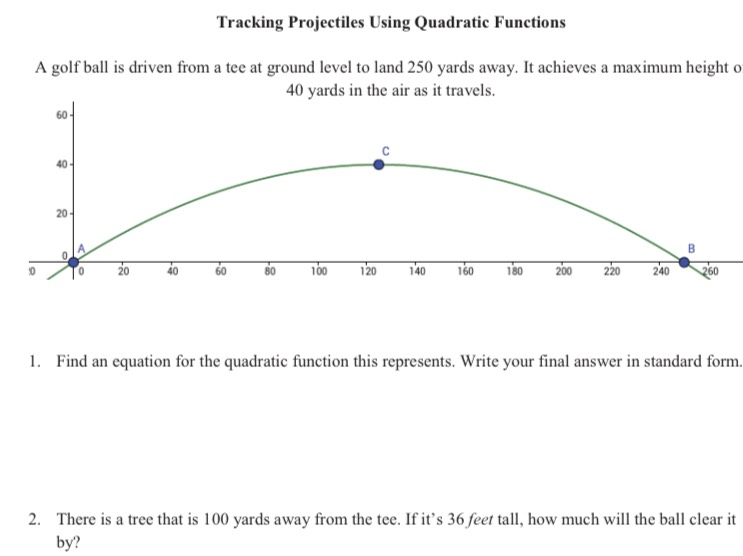### ¿Todavía tienes preguntas de matemáticas?

Pregunte a nuestros tutores expertos
Algebra
PreguntaA golf ball is driven from a tee at ground level to land $$250$$ yards away. It achieves a maximum height  $$40$$ yards in the air as it travels.

1. Find an equation for the quadratic function this represents. Write your final answer in standard form. 2. There is a tree that is $$100$$ yards away from the tee. If it's $$36$$ feet tall, how much will the ball clear it by?

$$y= -\frac{1}{390.625}\left(x- 125\right)^{2}+ 40$$